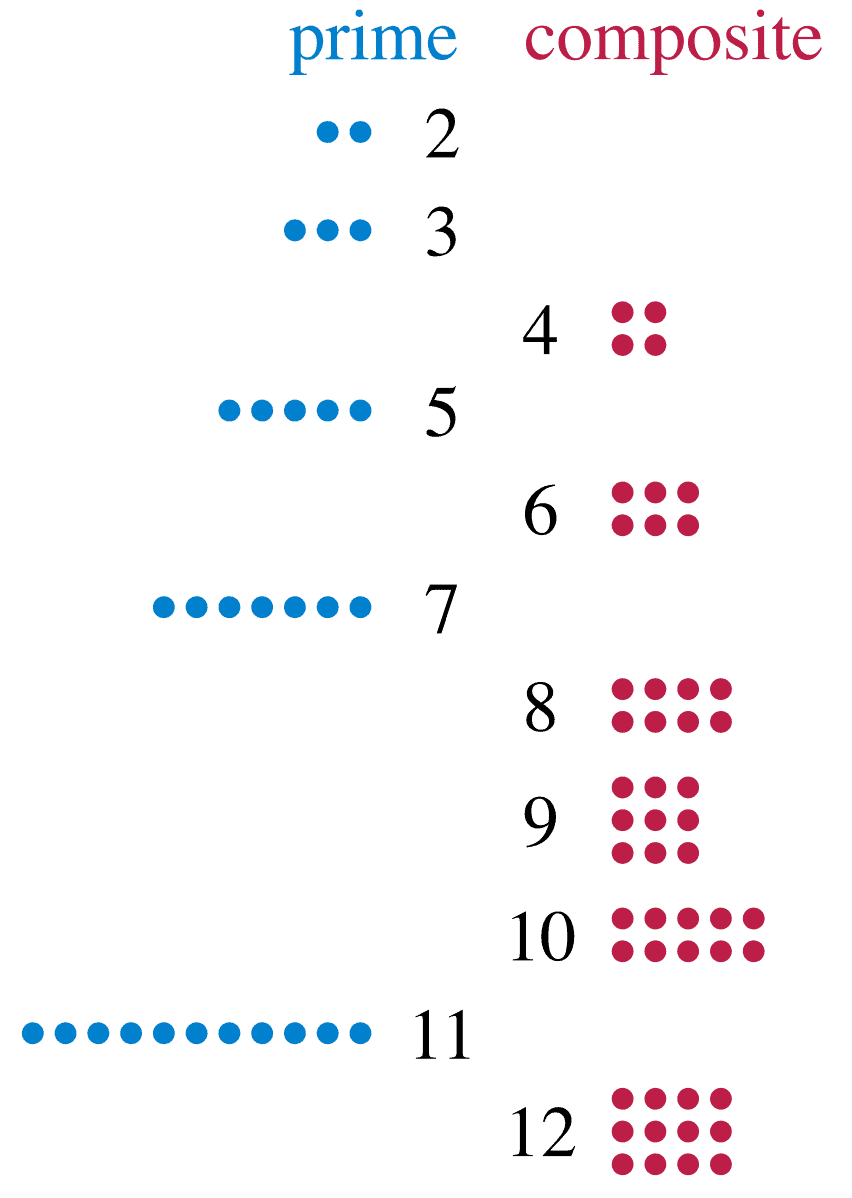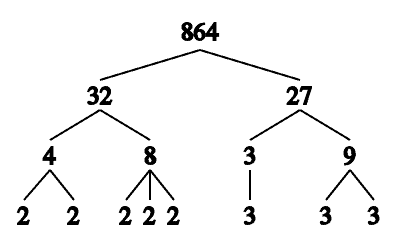# How Many Prime Numbers Are There? (3 Key Concepts)

Prime numbers are the building blocks of integers, and they are important in number theory and cryptography.  There are lots of prime numbers that we know of, but this still leaves the question of how numerous they are.

So, how many prime numbers are there?  There are infinitely many prime numbers, and we can never run out of prime numbers. Also, there is no largest prime number – they grow without bound. There are 4 primes between 1 and 10, 8 primes between 1 and 20, 25 primes between 1 and 100, and 168 primes between 1 and 1000.

Of course, there are also twin primes (such as the pair 3 and 5) to consider – there may be infinitely many such pairs, but this is still unproven.

In this article, we’ll talk about prime numbers and how to prove that there are infinitely many.  We’ll also look at some examples of how to find all of the primes between 1 and N.

Let’s get started.

## How Many Prime Numbers Are There?

There are infinitely many prime numbers.  That is, no matter how many prime numbers we write out in a list, we can always find more to add to the list.There are infinitely many prime numbers. However, as we look at larger numbers, primes are rare compared to composites.

To show this, we will use proof by contradiction.  Assume that there are only finitely many prime numbers, and write them in a list, labeling them with subscripts from 1 to N:

• p1, p2, … , pN

Then, take the product P of all N prime numbers on our list:

• P = p1 x p2 x … x pN

Finally, add 1 to this number to get P + 1.

Since P is a product of every prime number in our list, then P + 1 is larger than any number on the list.

Thus, P + 1 is not on the list, and so it is not prime.  Since P + 1 is not prime, it must be composite.

Therefore, it is the product of prime numbers from our list.  This means that there must be some prime number pi in our list so that P + 1 divided by pi has a zero remainder.

However, P + 1 divided by any prime in our list will have a remainder of 1, since:

• (P + 1) / p1 = (p1 x p2 x … x pN + 1) / p1 = p2 x … x pN + 1 / p1
• (P + 1) / p2 = (p1 x p2 x … x pN + 1) / p2 = p1 x p3 x … x pN + 1 / p2
• (P + 1) / pN = (p1 x p2 x … x pN + 1) / pN = p1 x p2 x … x pN-1 + 1 / pN

No matter which prime pi we divide by, we always get a remainder of 1.  So, P + 1 is not divisible by any prime on our list.

This implies that either:

• P + 1 is prime, which is a contradiction (we said P + 1 is not prime), or
• P + 1 is composite and is a product of other primes that were not on our list, which is also a contradiction (we said our list contained all prime numbers).

So, there must be infinitely many prime numbers.  Thus, we can never run out of prime numbers.

Note: due to the Fundamental Theorem of Arithmetic, it is also true that every integer greater than 1 is either prime or has a unique prime factorization.  That means that there is only one combination of prime numbers (raised to the proper powers) that will result in a given integer product.

### How Many Prime Numbers Are Between 1 & N?

To find out how many prime numbers are between 1 and N, we can use a method called the Sieve of Eratosthenes.

Basically, we are using the process of elimination to remove composite numbers from our list until only prime numbers remain.

Here are the steps for using the Sieve of Eratosthenes:

• First, list out the numbers from 2 to N in a grid.
• Next, cross out all of the multiples of 2 (that is, even numbers, or those divisible by 2 with no remainder – anything ending in 0, 2, 4, 6, or 8).
• Then, cross out all of the multiples of 3 (that is, numbers divisible by 3 with no remainder).
• Continue in this way by crossing out all of the multiples of 5, 7, etc.
• Only the prime numbers will remain in the final list.

Let’s look at an example of how to use the Sieve.

### How Many Prime Numbers Are There Between 1 & 10?

Using the Sieve of Eratosthenes, we list out the numbers 1 to 10 in a grid:

Next, we cross out the numbers that are multiples of 2 (except 2, which is prime):

Next, we cross out the numbers that are multiples of 3 (except 3, which is prime):

The remaining numbers in the list are prime.

So, there are 4 prime numbers between 1 and 10: 2, 3, 5, and 7.  The other numbers between 1 and 10 are composite, since:

• 4 = 2*2 = 22
• 6 = 2*3
• 8 = 2*2*2 = 23
• 9 = 3*3 = 32
• 10 = 2*5

### How Many Prime Numbers Are There Between 1 & 20?

There are 8 prime numbers between 1 and 20: 2, 3, 5, 7, 11, 13, 17, and 19.  The other numbers between 1 and 20 are composite, since:

• 12 = 2*1*3 = 22*3
• 14 = 2*7
• 15 = 3*5
• 16 = 2*2*2*2 = 24
• 18 = 2*3*3 = 2*32
• 20 = 2*2*5 = 22*5

### How Many Prime Numbers Are There Between 1 & 100?

There are 25 prime numbers between 1 and 100:

• 2
• 3
• 5
• 7
• 11
• 13
• 17
• 19
• 23
• 29
• 31
• 37
• 41
• 43
• 47
• 53
• 59
• 61
• 67
• 71
• 73
• 79
• 83
• 89
• 97

### How Many Prime Numbers Are There Between 1 & 1000?

There are 168 prime numbers between 1 and 1000.  The largest prime number less than 1000 is 997.

### How Many Prime Numbers Are Even?

There is only one even prime number: 2.  We can prove this as follows:

• 2 is even, since we can write it as 2 = 2*1.  2 is also prime, since it only has 1 and itself as factors.
• Any other positive even number E has the form E = 2N, where N is an integer greater than 1.  Since E has factors of 1, 2, and N for N > 1, then E is composite (not prime).

So, no other even number besides 2 is prime.  Thus, 2 is the only even prime number.

Another way to say this is that 2 is the only prime number divisible by 2.  Every prime number other than 2 is an odd prime.

Similarly, 3 is the only prime number divisible by 3, and 5 is the only prime number divisible by 5, and so forth.

### What Are Twin Primes?

A pair of twin primes P and P + 2 are both prime and are only 2 units apart (that is, one is 2 greater than the other).

Some examples of twin primes are:

• 3 and 5
• 5 and 7
• 11 and 13
• 17 and 19
• 29 and 31
• 41 and 43

The twin prime conjecture suggests that there are infinitely many pairs of twin primes.  However, this remains to be proven.

### How Many Numbers Between 1 & N Are Relatively Prime To N?

Two integers M and N are relatively prime (also called coprime or mutually prime) if the only common factor of M and N is 1.  In other words, M and N are relatively prime if they share no common prime factor.

For example, 10 and 21 are relatively prime, since 10 = 2*5 and 21 = 3*7.  Since there is no overlap between {2, 5} and {3, 7}, 10 and 21 are relatively prime.Find the prime factors of two numbers and compare the list of primes. If there is nothing in common, then they are relatively prime.

Note that two distinct prime numbers are automatically relatively prime.  Also, every number from 1 to P – 1 is relatively prime to a prime number P.

If we want to find out how many numbers are relatively prime to N, we can use Euler’s totient function (or Euler’s phi function).

For a prime number P, there are P – 1 positive integers that are relatively prime to P (the numbers 1, 2, … , P – 1).

## Conclusion

Now you know how many prime numbers there are (infinitely many!) and a little more about how to come to this conclusion.